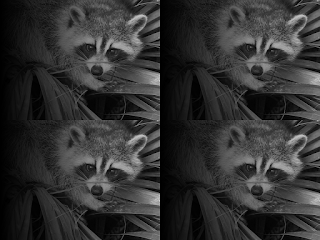## Solving edge-effects when blurring

One of the problems with blurring an image is what happens at the edges. For example, consider an image that has a large brightness difference between the left and right edges:A common way to blur an image is to take advantage of the convolution theorem and turn a Gaussian blur (convolution of the source image with a Gaussian peak) into a multiplication in the Fourier domain (convert src and Gaussian peaks into their Fourier representations, multiply instead of convolve, then inverse Fourier transform). Here's some code to do just that:

``````
import numpy as np
import cv2

"""
Return a gaussian of the given shape, with the given standard deviations.
Treats 0,0 as origin
"""
return reduce(np.multiply,
(np.exp(-dx**2 / (2*sd**2))
for sd, dx in zip(sds, np.indices(shape))))

def mirror_image(im):
"""
Takes an image A, and mirrors in x and y
"""
out = im
left = np.vstack([im, im[::-1, :]])
return np.hstack([left, left[:, ::-1]])

def gaussian_blur_cv2_dft(im, amount):
# gaussian blur using dft
h, w = im.shape
[h/(2*np.pi*amount), w/(2*np.pi*amount)])
full_gauss = mirror_image(quarter_gauss)
Fsrc = cv2.dft(im.astype(float), None, cv2.DFT_COMPLEX_OUTPUT)
Fsrc[..., 0] *= full_gauss
Fsrc[..., 1] *= full_gauss
inv = cv2.dft(Fsrc, None, cv2.DFT_INVERSE | cv2.DFT_REAL_OUTPUT | cv2.DFT_SCALE)
return inv.astype(im.dtype)
``````

This uses the opencv dft (discrete fourier transform) method to perform the transformations to and from Fourier space (there's no need to actually Fourier transform the Gaussian peak, as the transform is just another Gaussian. We just need to work out how big the peak is in Fourier space and directly create it with that size).

If we do this with amount=50, we get the following (for the blurred images below, I've scaled by 2 to exaggerate the effects)The problem with this is that the left edge has a bright spot that doesn't appear in the original, and the right hand edge is darker than it should be. This is because of the implicit boundary conditions of the discrete-Fourier transform. The images is treated as if it is repeated periodically:And we can see why blurring this produces 'artefacts' on the edges.

### Solution #1: Mirroring the image

One solution is to simply mirror the image, blur it, and then crop it again. The mirrored image before blurring looks like:

Then, after blurring and cropping, we get:

This looks a lot better than our first attempt. The problem is that it uses 4 times more memory. Here's the code to do this. It uses exactly the same function as before

``````
src_h, src_w = src.shape
src_x4 = mirror_image(src)
dft_x4_blur = gaussian_blur_cv2_dft(src_x4, blur_amount)
dft_x4_blur_cropped = dft_x4_blur[:src_h, :src_w]
``````

### Solution #2: DCT

The problem with the discrete Fourier transform method is purely that its boundary conditions are perfect for repeating data, but don't work when blurring images: we'd prefer a symmetric boundary condition (effectively mirroring the image for us, like we did above). Luckily, there's a transform with exactly this property: the discrete cosine transform. Using this instead of a Fourier transform actually makes things a bit easier (the data is real all the way through). Here's the code for a dct-based blurring function:

``````
def gaussian_blur_cv2_dct(im, amount):
# gaussian blur using cv2 dct
h, w = im.shape
[h/(np.pi*amount), w/(np.pi*amount)])
Fsrc = cv2.dct(im.astype(float))
Fsrc *= gaussian
inv = cv2.dct(Fsrc, None, cv2.DCT_INVERSE)
return inv.astype(im.dtype)
``````

Here's the results using this:Again, this has solved the edge artifact issues, and this time we didn't need to use four times the data.

### Performance

dft blur: 50ms
dft scaled then cropped: 229ms
dct blur: 87ms

I was kind of hoping that not only would the dct work better than the dft, but that it would also be significantly faster (it is limited to purely real data, and is used all the time in decoding JPEGs (and I expect movie files too) so I was hoping it would be massively optimized). But the opencv2 version runs significantly slower than the dft. It's still significantly faster than the scaling, blurring, then cropping approach though, and I expect uses a lot less memory.

The Jupyter notebook I used to perform all these experiments is on GitHub.